Function to plot 3D graphics or image and/or contour plots for bivariate effects/functions.

plot3d(x, residuals = FALSE, col.surface = NULL,
ncol = 99L, swap = FALSE, col.residuals = NULL, col.contour = NULL,
c.select = NULL, grid = 30L, image = FALSE, contour = FALSE,
legend = TRUE, cex.legend = 1, breaks = NULL, range = NULL,
digits = 2L, d.persp = 1L, r.persp = sqrt(3), outscale = 0,
data = NULL, sep = "", shift = NULL, trans = NULL,
type = "mba", linear = FALSE, extrap = FALSE,
k = 40, ...)

## Arguments

x

A matrix or data frame, containing the covariates for which the effect should be plotted in the first and second column and at least a third column containing the effect. Another possibility is to specify the plot via a formula, e.g. for simple plotting of bivariate surfaces z ~ x + y, see the examples. x may also be a character file path to the data to be used for plotting.

residuals

If set to TRUE, residuals may also be plotted if available. Residuals must be supplied as an attribute named "residuals", which is a matrix or data frame where the first two columns are covariates and the third column the residuals.

col.surface

The color of the surface, may also be a function, e.g. col.surface = heat.colors.

ncol

the number of different colors that should be generated, if col.surface is a function.

swap

If set to TRUE colors will be represented in reverse order.

col.residuals

The color of the partial residuals, or if contour = TRUE the color of the contour lines.

col.contour

The color of the contour lines.

c.select

Integer vector of maximum length of columns of x, selects the columns of the resulting data matrix that should be used for plotting. E.g. if x has 5 columns, then c.select = c(1, 2, 5) will select column 1, 2 and 5 for plotting. If c.select = 95 or c.select = 80, function plot3d will search for the corresponding columns to plot a 95$$\%$$ or 80$$\%$$ confidence surfaces respectively. Note that if e.g. c.select = c(1, 2), plot3d will use columns 1 + 2 and 2 + 2 for plotting.

grid

The grid size of the surface(s).

image

If set to TRUE, an image.plot is drawn.

contour

If set to TRUE, a contour plot is drawn.

legend

If image = TRUE an additional legend may be added to the plot.

cex.legend

The expansion factor for the legend text, see text.

breaks

A set of breakpoints for the colors: must give one more breakpoint than ncol.

range

Specifies a certain range values should be plotted for.

digits

Specifies the legend decimal places.

d.persp

See argument d in function persp.

r.persp

See argument r in function persp.

outscale

Scales the outer ranges of x and z limits used for interpolation.

data

If x is a formula, a data.frame or list. By default the variables are taken from environment(x): typically the environment from which plot3d is called. Note that data may also be a character file path to the data.

sep

The field separator character when x or data is a character, see function read.table.

shift

Numeric constant to be added to the smooth before plotting.

trans

Function to be applied to the smooth before plotting, e.g., to transform the plot to the response scale.

type

Character, which type of interpolation method should be used. The default is type = "akima", see function interp. The two other options are type = "mba", which calls function mba.surf of package MBA, or type = "mgcv", which uses a spatial smoother withing package mgcv for interpolation. The last option is definitely the slowest, since a full regression model needs to be estimated.

linear

Logical, should linear interpolation be used withing function interp?

extrap

Logical, should interpolations be computed outside the observation area (i.e., extrapolated)?

k

Integer, the number of basis functions to be used to compute the interpolated surface when type = "mgcv".

...

Parameters passed to colorlegend if an image plot with legend is drawn, also other graphical parameters, please see the details.

## Details

For 3D plots the following graphical parameters may be specified additionally:

• cex: Specify the size of partial residuals,

• col: It is possible to specify the color for the surfaces if se > 0, then e.g. col = c("green", "black", "red"),

• pch: The plotting character of the partial residuals,

• ...: Other graphical parameters passed functions persp, image.plot and contour.

## Note

Function plot3d can use the akima package to construct smooth interpolated surfaces, therefore, package akima needs to be installed. The akima package has an ACM license that restricts applications to non-commercial usage, see

Function plot3d prints a note referring to the ACM license. This note can be suppressed by setting

options("use.akima" = TRUE)

colorlegend, plot2d, plotmap, plotblock, sliceplot.

## Examples

## Generate some data.
set.seed(111)
n <- 500

## Regressors.
d <- data.frame(z = runif(n, -3, 3), w = runif(n, 0, 6))

## Response.
d$y <- with(d, 1.5 + cos(z) * sin(w) + rnorm(n, sd = 0.6)) if (FALSE) ## Estimate model. b <- bamlss(y ~ s(z,w), data = d) summary(b) #> Error in summary(b): object 'b' not found ## Plot estimated effect. plot(b, model = "mu", term = "s(z,w)") #> Error in h(simpleError(msg, call)): error in evaluating the argument 'x' in selecting a method for function 'plot': object 'b' not found ## Extract fitted values. f <- fitted(b, model = "mu", term = "s(z,w)", intercept = FALSE) #> Error in fitted(b, model = "mu", term = "s(z,w)", intercept = FALSE): object 'b' not found f <- cbind(d[, c("z", "w")], f) #> Error in data.frame(..., check.names = FALSE): object 'f' not found ## Now use plot3d(). plot3d(f) #> Error in plot3d(f): object 'f' not found plot3d(f, swap = TRUE) #> Error in plot3d(f, swap = TRUE): object 'f' not found plot3d(f, grid = 100, border = NA) #> Error in plot3d(f, grid = 100, border = NA): object 'f' not found ## Only works if columns are named with ## '2.5 plot3d(f, c.select = 95, border = c("red", NA, "green"), col.surface = c(1, NA, 1), resid = TRUE, cex.resid = 0.2) #> Error in plot3d(f, c.select = 95, border = c("red", NA, "green"), col.surface = c(1, NA, 1), resid = TRUE, cex.resid = 0.2): object 'f' not found ## Now some image and contour. # plot3d(f, image = TRUE, legend = FALSE) # plot3d(f, image = TRUE, legend = TRUE) # plot3d(f, image = TRUE, contour = TRUE) # plot3d(f, image = TRUE, contour = TRUE, swap = TRUE) # plot3d(f, image = TRUE, contour = TRUE, col.contour = "white") # plot3d(f, contour = TRUE) # plot3d(f, image = TRUE, contour = TRUE, c.select = 3) # plot3d(f, image = TRUE, contour = TRUE, c.select = "Mean") # plot3d(f, image = TRUE, contour = TRUE, c.select = "97.5 ## Variations. d$f1 <- with(d, sin(z) * cos(w))
with(d, plot3d(cbind(z, w, f1)))

## Same with formula.
plot3d(sin(z) * cos(w) ~ z + w, zlab = "f(z,w)", data = d)plot3d(sin(z) * cos(w) ~ z + w, zlab = "f(z,w)", data = d,
ticktype = "detailed")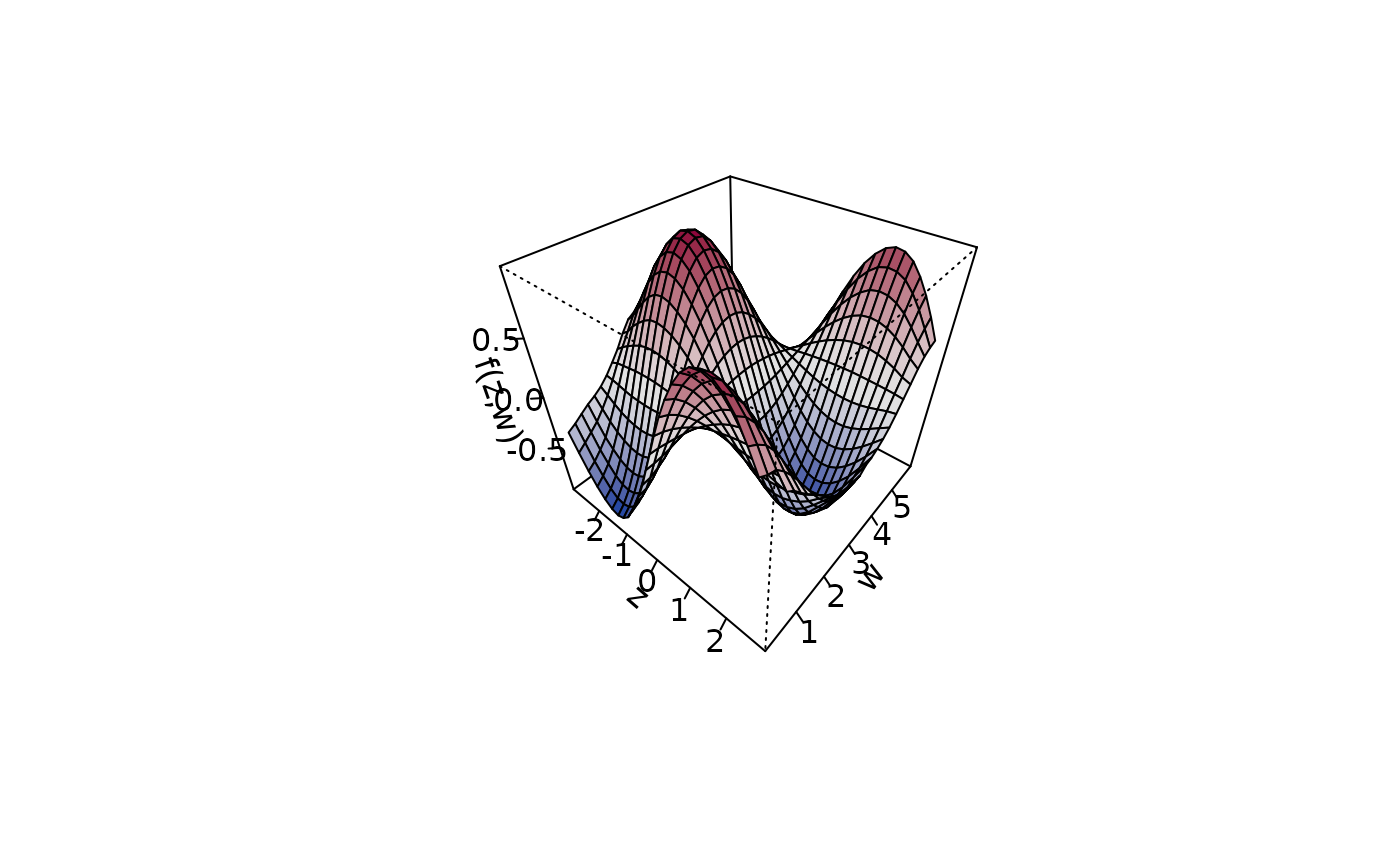## Play with palettes.
plot3d(sin(z) * cos(w) ~ z + w, col.surface = heat.colors, data = d)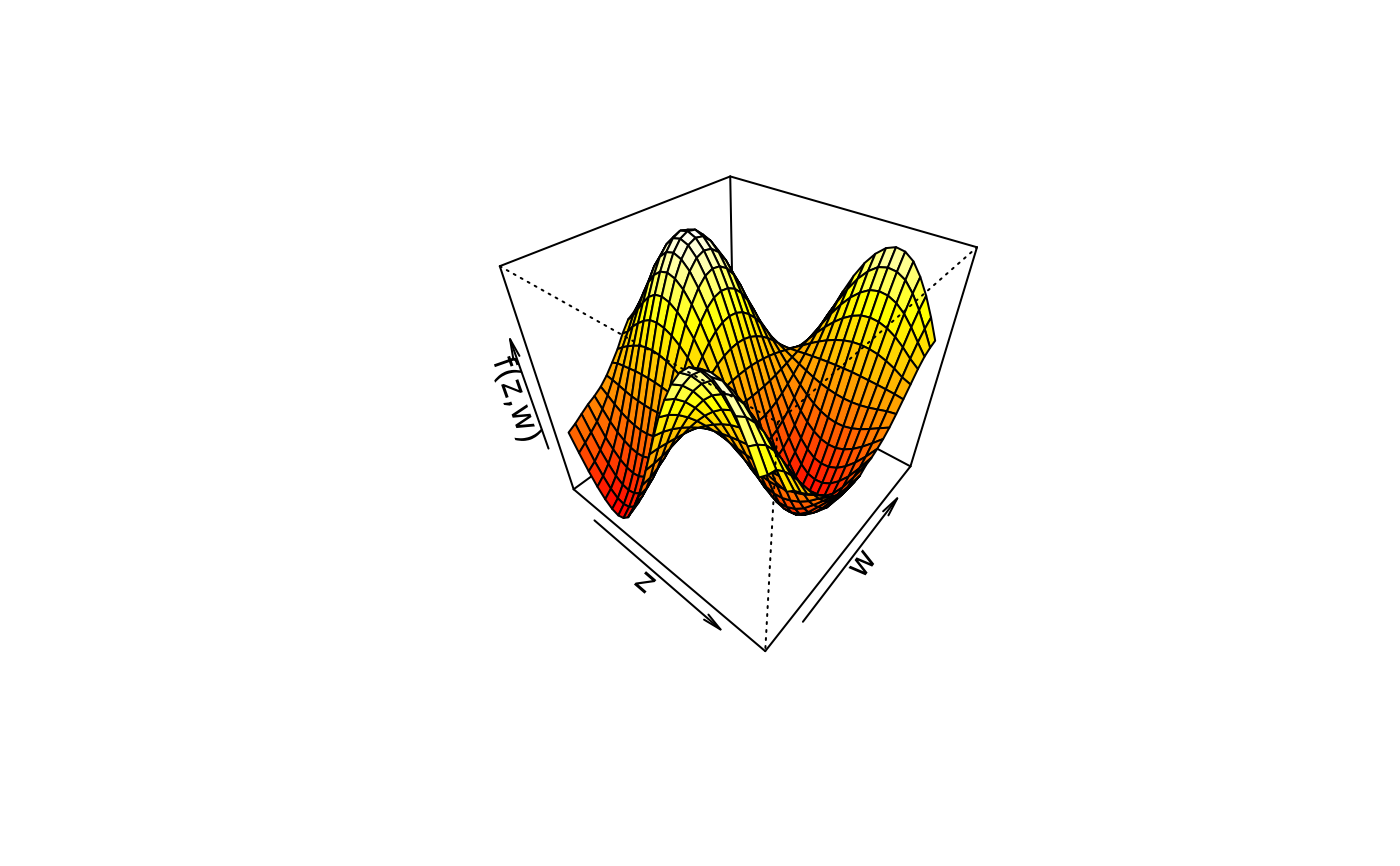plot3d(sin(z) * cos(w) ~ z + w, col.surface = topo.colors, data = d)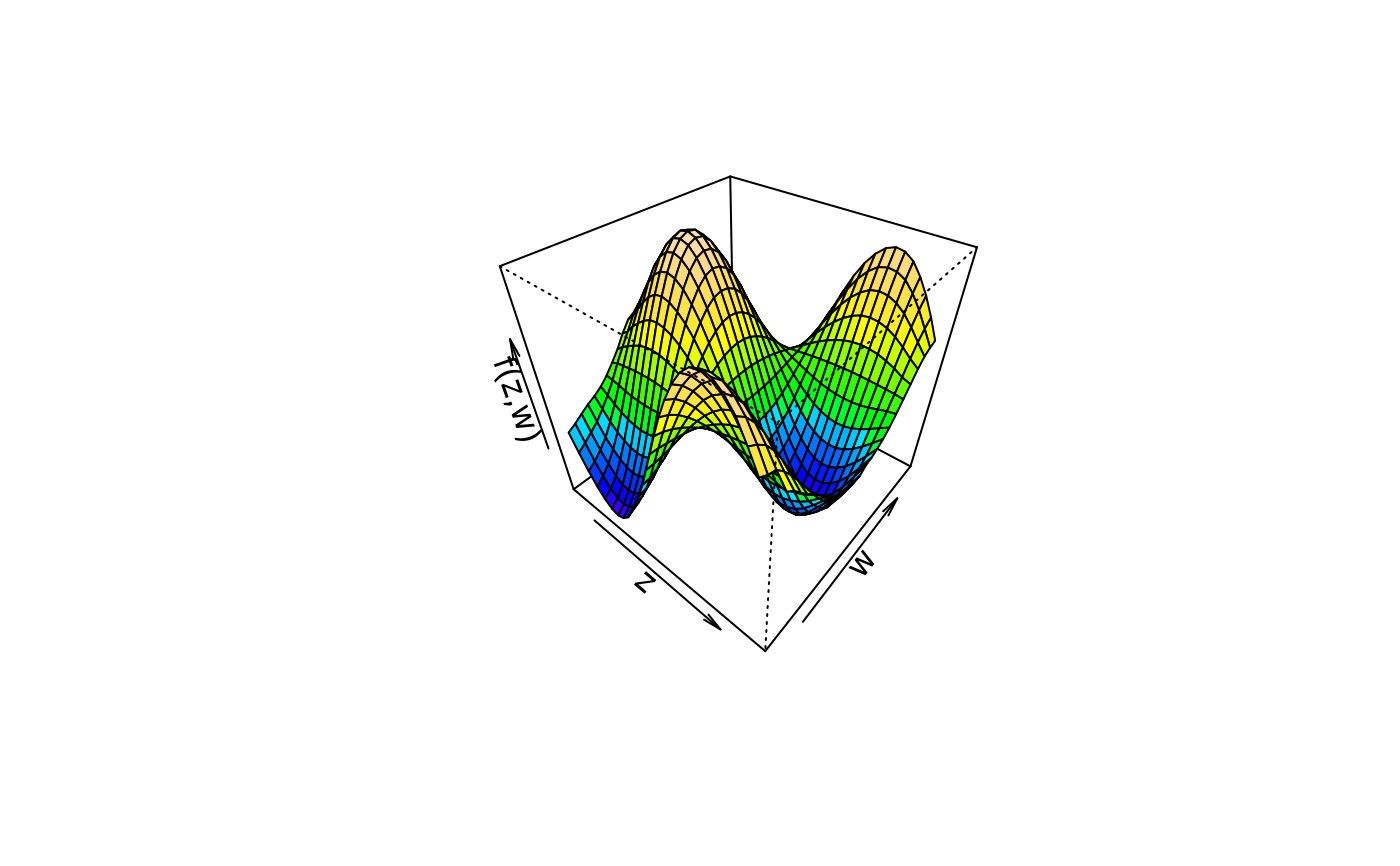plot3d(sin(z) * cos(w) ~ z + w, col.surface = cm.colors, data = d)plot3d(sin(z) * cos(w) ~ z + w, col.surface = rainbow, data = d)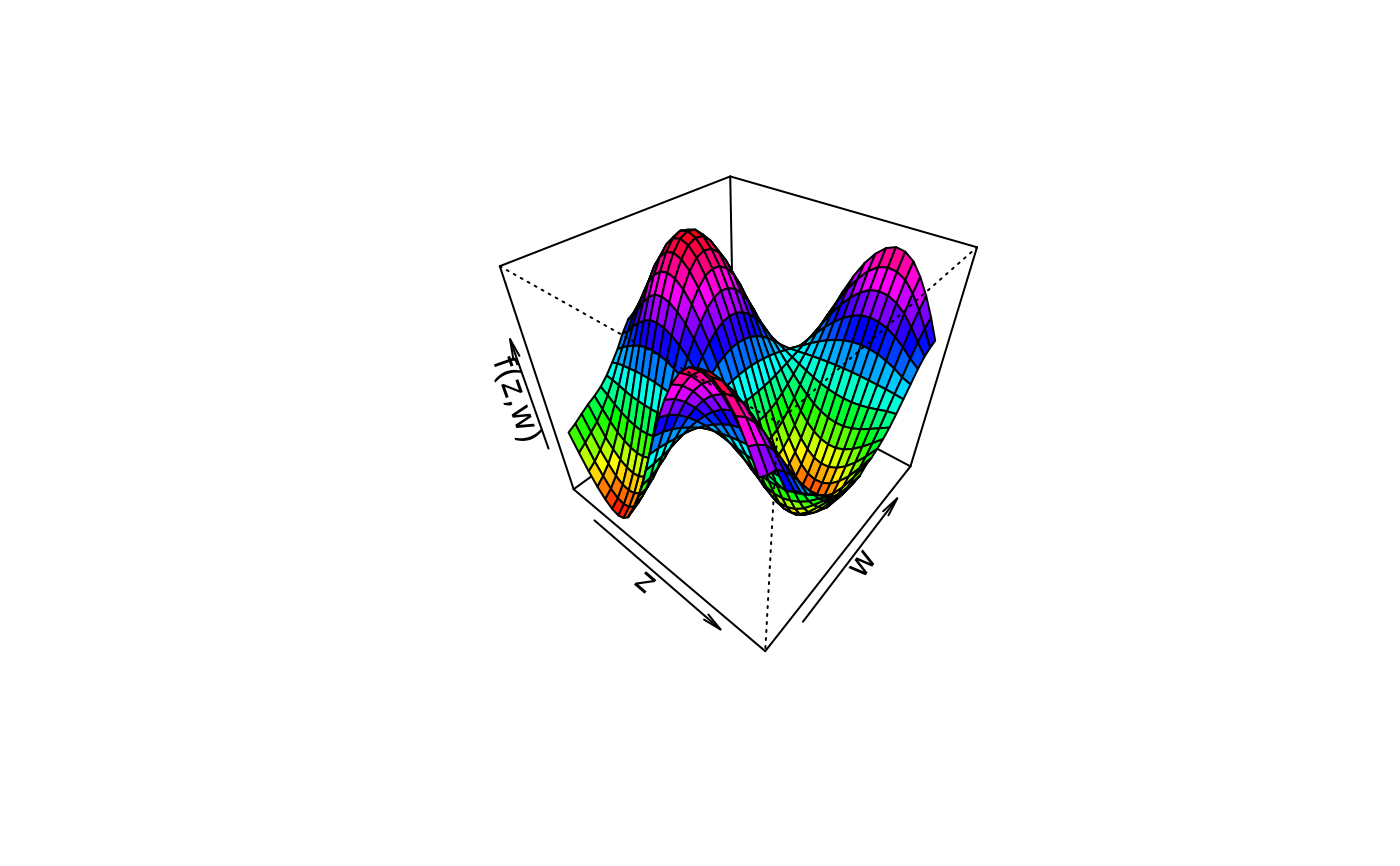plot3d(sin(z) * cos(w) ~ z + w, col.surface = terrain.colors, data = d)plot3d(sin(z) * cos(w) ~ z + w, col.surface = rainbow_hcl, data = d)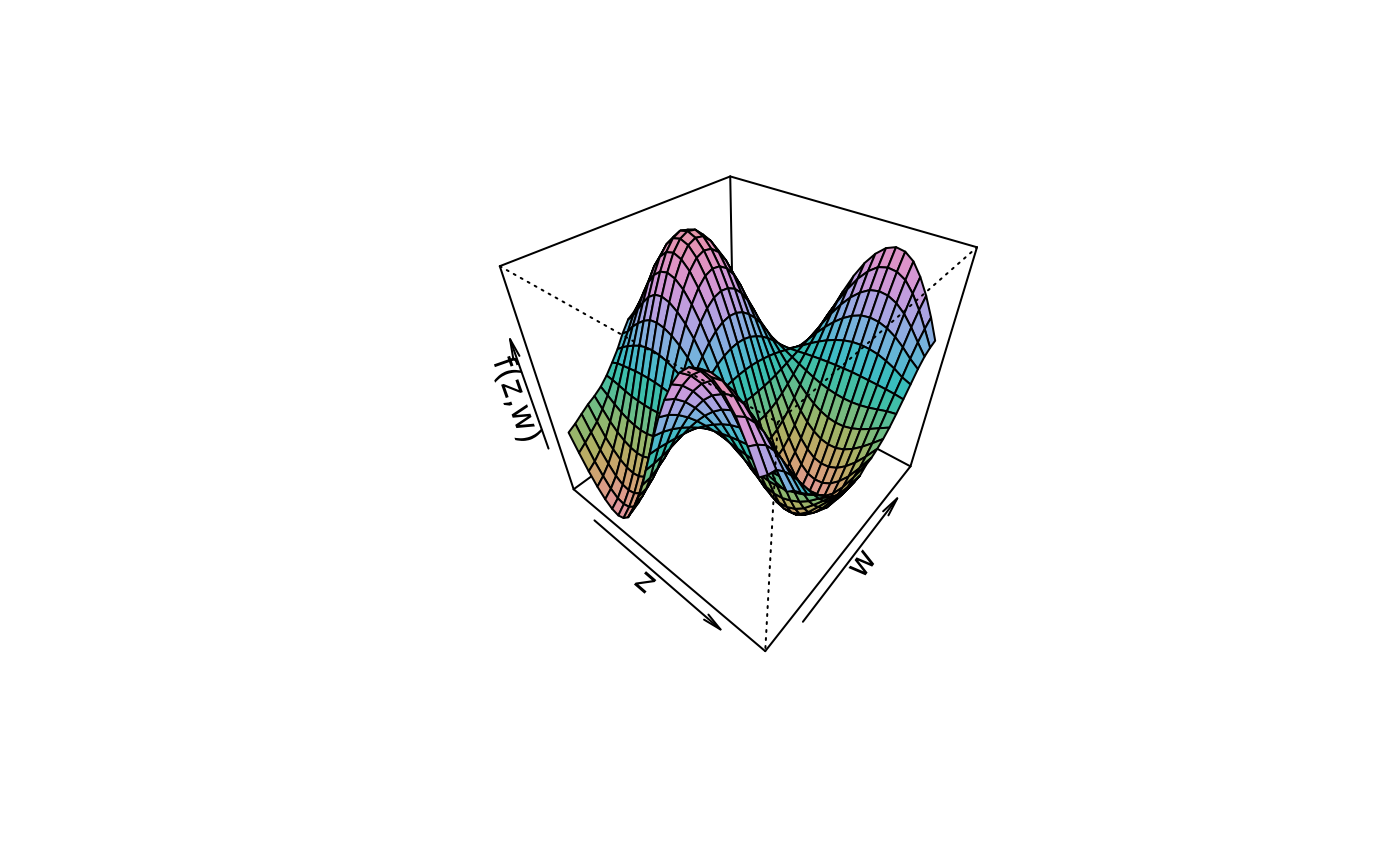plot3d(sin(z) * cos(w) ~ z + w, col.surface = diverge_hcl, data = d)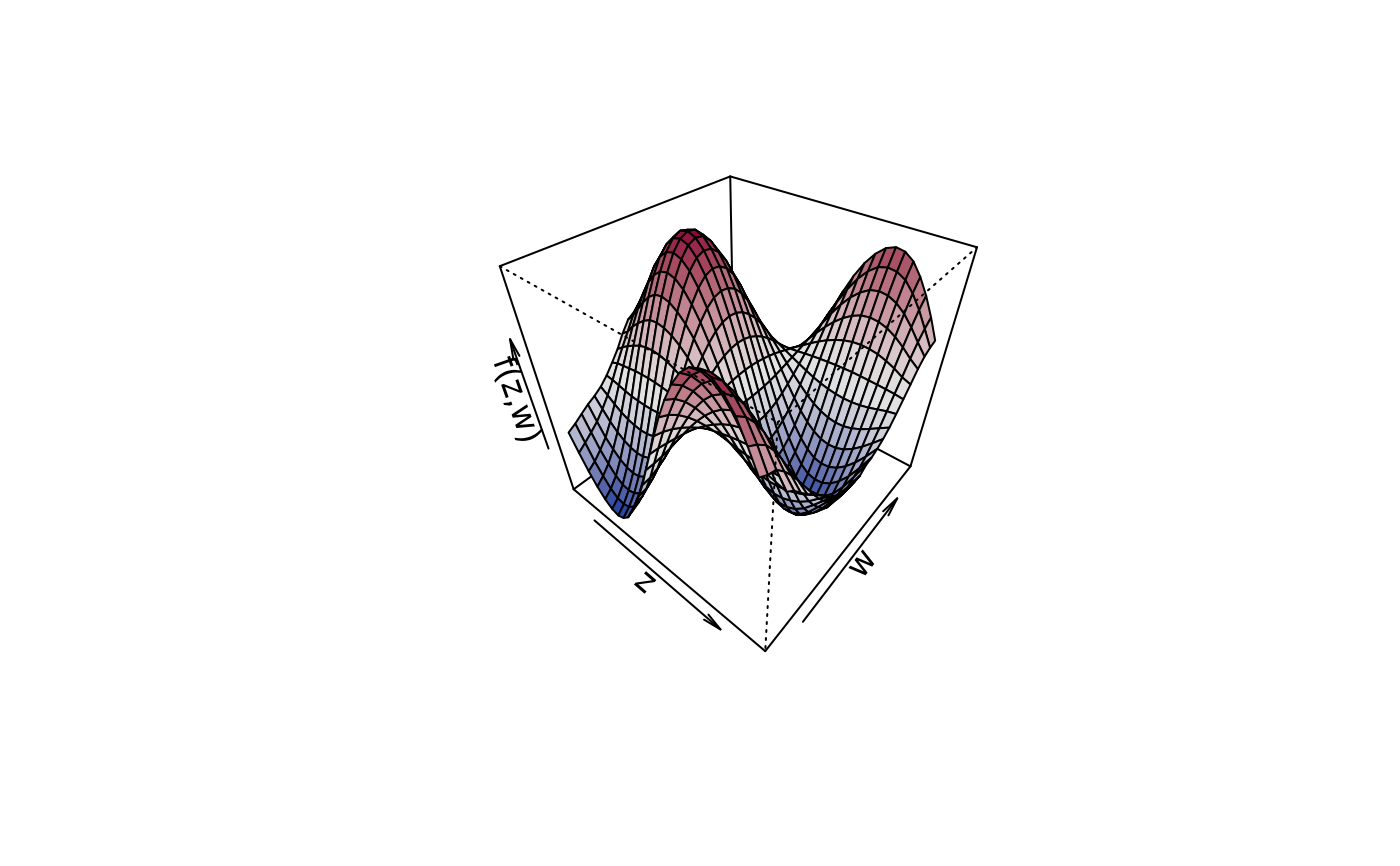plot3d(sin(z) * cos(w) ~ z + w, col.surface = sequential_hcl, data = d)plot3d(sin(z) * cos(w) ~ z + w,
col.surface = rainbow_hcl(n = 99, c = 300, l = 80, start = 0, end = 100),
data = d)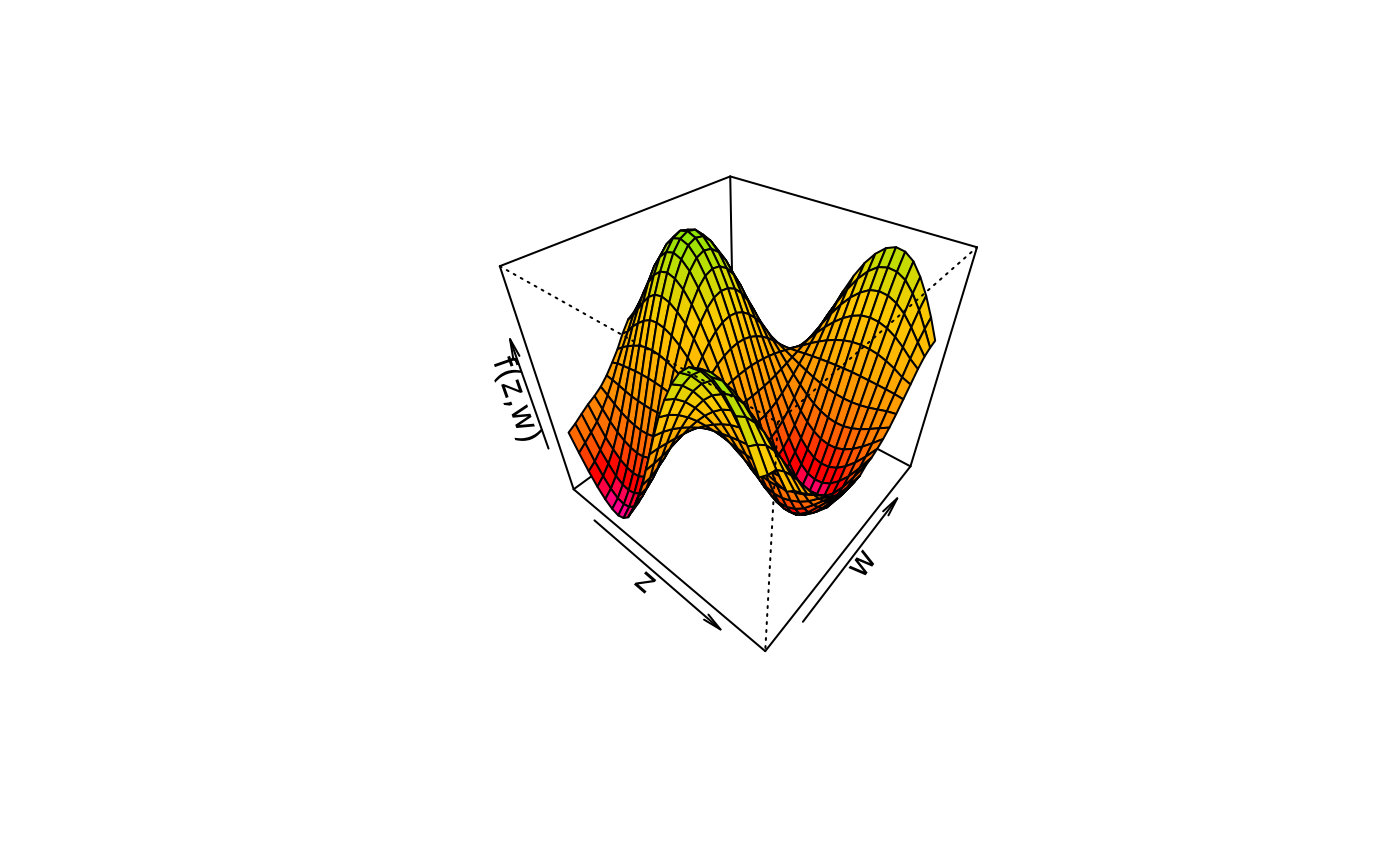# plot3d(sin(z) * cos(w) ~ z + w,
#  col.surface = rainbow_hcl(n = 99, c = 300, l = 80, start = 0, end = 100),
#  image = TRUE, grid = 200, data = d)